This fact should be plausible, and can be proved by a straightforward calculation as follows.

Parametrize the 2-sphere S2 of radius 1 by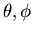(longitude,co-latitude). Then the usual metric is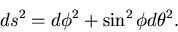Note that the curves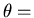constant are great circles.

Parametrize C= the cone on S2 bywhere r is the distance from the cone point. Then the usual metric on C (with the cone point at distance k from the sphere) is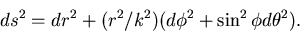A curvewill have coordinatesfor t in [0,1]. If the curve is differentiable, its length isSuppose the curve joins two points in the cone over the great circle, i.e. that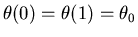. Then the curve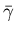given by, which lies entirely in the cone over the great circle, will have same endpoints as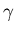and will be shorter, since all the terms in the length integral were positive, and the term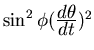will now be zero.

The path of a light ray is the curve of shortest length between its endpoints. So ifis the path of a light ray between two points in the cone over a great circle, thenmust lie entirely in that cone.

Back to Gravitational Lensing and Geometric Lensing.

Tony Phillips
1999-01-23